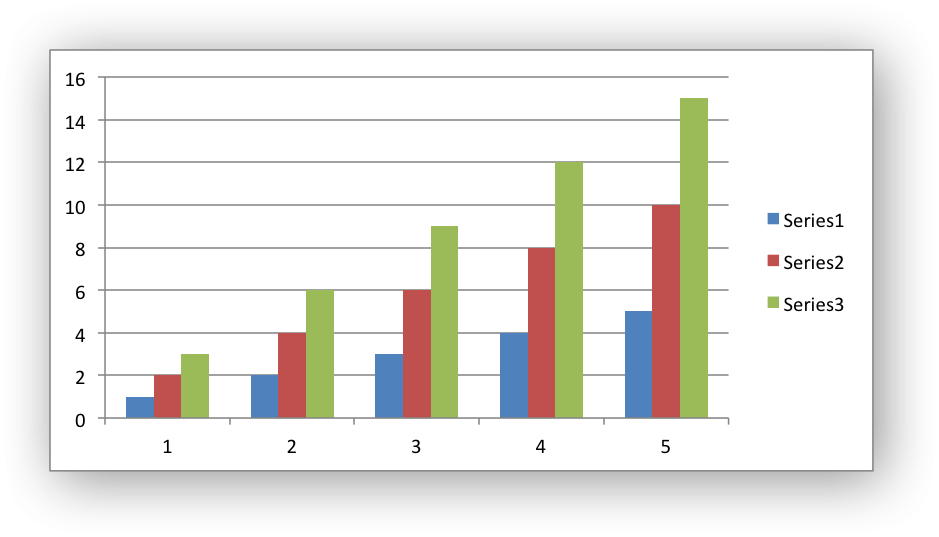libxlsxwriter
chart.c

An example of creating a simple column chart with 3 data series./*
* An example of a simple Excel chart using the libxlsxwriter library.
*
* Copyright 2014-2021, John McNamara, jmcnamara@cpan.org
*
*/
#include "xlsxwriter.h"
/* Write some data to the worksheet. */
void write_worksheet_data(lxw_worksheet *worksheet) {
uint8_t data = {
/* Three columns of data. */
{1, 2, 3},
{2, 4, 6},
{3, 6, 9},
{4, 8, 12},
{5, 10, 15}
};
int row, col;
for (row = 0; row < 5; row++)
for (col = 0; col < 3; col++)
worksheet_write_number(worksheet, row, col, data[row][col], NULL);
}
/* Create a worksheet with a chart. */
int main() {
lxw_workbook *workbook = workbook_new("chart.xlsx");
/* Write some data for the chart. */
write_worksheet_data(worksheet);
/* Create a chart object. */
/* Configure the chart. In simplest case we just add some value data
* series. The NULL categories will default to 1 to 5 like in Excel.
*/
chart_title_set_name(chart, "Year End Results");
/* Insert the chart into the worksheet. */
worksheet_insert_chart(worksheet, CELL("B7"), chart);
return workbook_close(workbook);
}
workbook_close
lxw_error workbook_close(lxw_workbook *workbook)
Close the Workbook object and write the XLSX file.
lxw_chart_series * chart_add_series(lxw_chart *chart, const char *categories, const char *values)
Add a data series to a chart.
workbook_new
lxw_workbook * workbook_new(const char *filename)
Create a new workbook object.
chart_title_set_name_font
void chart_title_set_name_font(lxw_chart *chart, lxw_chart_font *font)
Set the font properties for a chart title.
lxw_chart_font
Struct to represent a chart font.
Definition: chart.h:699
lxw_worksheet
Struct to represent an Excel worksheet.
Definition: worksheet.h:2107
lxw_chart
Struct to represent an Excel chart.
Definition: chart.h:1090
worksheet_insert_chart
lxw_error worksheet_insert_chart(lxw_worksheet *worksheet, lxw_row_t row, lxw_col_t col, lxw_chart *chart)
Insert a chart object into a worksheet.
LXW_EXPLICIT_FALSE
@ LXW_EXPLICIT_FALSE
Definition: common.h:56
lxw_workbook
Struct to represent an Excel workbook.
Definition: workbook.h:292
chart_title_set_name
void chart_title_set_name(lxw_chart *chart, const char *name)
Set the title of the chart.
LXW_COLOR_BLUE
@ LXW_COLOR_BLUE
Definition: format.h:184
lxw_chart_font::bold
uint8_t bold
Definition: chart.h:708
worksheet_write_number
lxw_error worksheet_write_number(lxw_worksheet *worksheet, lxw_row_t row, lxw_col_t col, double number, lxw_format *format)
Write a number to a worksheet cell.
CELL
#define CELL(cell)
Convert an Excel A1 cell string into a (row, col) pair.
Definition: utility.h:45